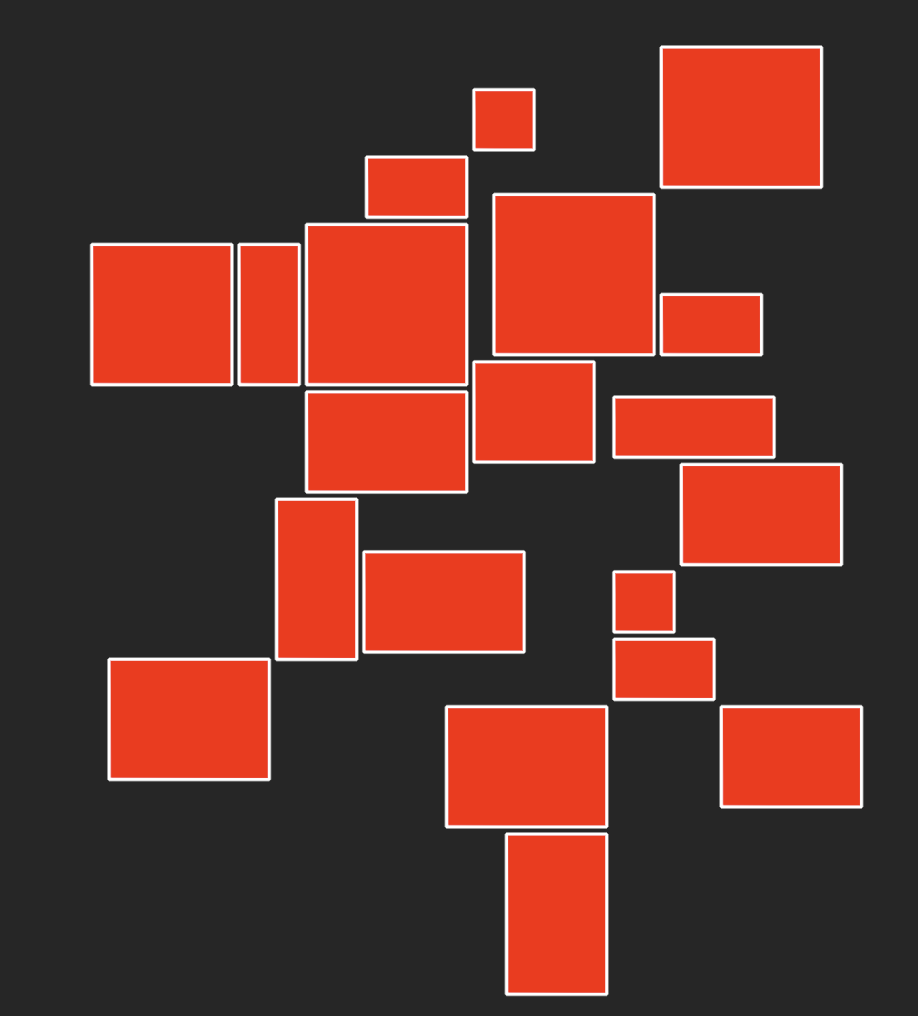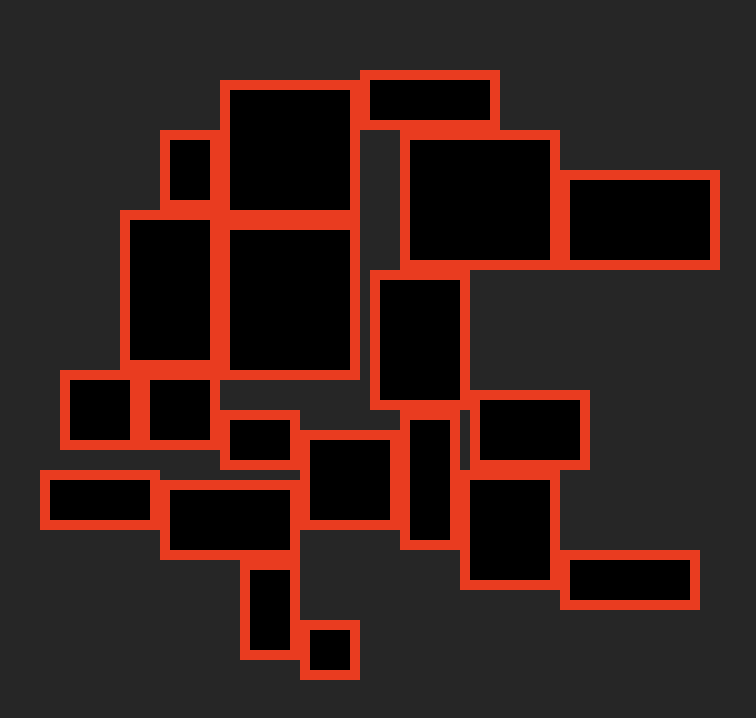# SF Rogue Room Arrangement

This article continues covering the challenges I faced while creating SF Rogue.

## Challenge 2

Now that we have our random rooms generated, we need to ensure that none of them overlap and where possible they should be placed adjacent to rooms they are close to, reducing the need for corridors.

We will continue using the same playground we created in the previous article, which can be found on GitHub.

Firstly we are going to add some Read-Only Computed Properties to the `Room` class, so that we can retrieve information easily from the drawn `node`.

``````    var frame: CGRect {
self.node.frame
}

var height: CGFloat {
self.node.frame.height
}

var width: CGFloat {
self.node.frame.width
}``````

As we are going to be moving overlapping rooms we also need a function that will allow us to change the position of the rooms `node` in the scene, add the following method after the properties you've just added...

``````public func moveTo(x: CGFloat = CGFloat.greatestFiniteMagnitude,
y: CGFloat = CGFloat.greatestFiniteMagnitude ) {
// Using 'fixed' default values means we only need to
// supply the values that need to change.
// If the default value is detected then the existing value is used

self.node.position = CGPoint(x: ( x == CGFloat.greatestFiniteMagnitude ? self.node.position.x : x ),
y: ( y == CGFloat.greatestFiniteMagnitude ? self.node.position.y : y ))
}
``````

Now that we can move the room, we need to work out which rooms are actually overlapping and if they are, move them. The current Playground places all the rooms in close proximity of each other, so as we move one room out of the way of the current room there's a possibility that the new position could overlap another room.

We need a way to manage continuous overlapping and an indication when there are no furhter overlaps. Looping through each room, comparing it to rooms that are below it in the "stack" of rooms in the array and moving rooms that overlap, makes the process manageable. It will also have the effect of fanning out the rooms (I hope).

Add the following function to the `Room` class...

``````
public func removeOverlap(rooms: [Room]) -> Int {
// Used to highlight that intersections were found
var intersectionCount = 0

// Pulling out values from the frame, for simplicity
let thisFrame = self.frame
let thisRoomBottom = thisFrame.minY
let thisRoomTop = thisFrame.maxY
let thisRoomLeft = thisFrame.minX
let thisRoomRight = thisFrame.maxX

for otherRoom in rooms {

if thisFrame.intersects(otherRoom.frame) {
// Check the frames and if they intersect the otherRoom needs to move

intersectionCount += 1

// In order to keep the "randomness" of the rooms,
// we calculate new X and Y positions based on this rooms position.
// Using the nextBool function we can get varying layouts

// The height and width are halved because the anchor point is in the centre of the room

let newY = randomSource.nextBool()
? thisRoomBottom - (otherRoom.height / 2)
: thisRoomTop + (otherRoom.height / 2)

let newX =  randomSource.nextBool()
? thisRoomLeft - (otherRoom.width / 2)
: thisRoomRight + (otherRoom.width / 2)

//Randomly pick which way to move the room
if randomSource.nextBool() {
otherRoom.moveTo(y: newY)
} else {
otherRoom.moveTo(x: newX)
}

if self.frame.intersects(otherRoom.frame) {
// they are still intersecting so change both values
otherRoom.moveTo(x: newX, y: newY)
}
}
}

return intersectionCount
}
``````

This method give us the ability at a `Room` level to ensure its not being overlapped by rooms that have been passed to it. All thats left is to call this from within the `GameScene`.

The above code uses a new class variable called `randomSource` to get some random true/false values. You'll need to add a private variable to the class...

``private let randomSource = GKRandomSource.sharedRandom()``

and an import of the `GameplayKit` to the top of the class

``import GameplayKit``

Open the main Playground code and add the following loop to the end of the `didMove` function...

``````
var arrangedCount = 0
repeat {
arrangedCount = arrangeRooms()
} while arrangedCount > 0
``````

This loop will repeat until we've been told there are no more overlapping rooms. Add the following function after the `didMove` function...

``````
func arrangeRooms() -> Int {
var overlappingCount = 0

// Loop through all the rooms, with the exception of the last one
for count in 0..<rooms.count-1 {

let thisRoom = rooms[count]

// Now remove the overlaps for this room and the rooms placed after it in the array
overlappingCount += thisRoom.removeOverlap(rooms: Array(rooms[count+1..<rooms.count]) )
}
return overlappingCount
}
``````

The Playground is now ready to run, and should produce something like this in the LiveView...What you'll probably notice is that although we've put the rooms next too each other in code, there appears to be gaps between them. This is being caused by the `lineWidth` thats been set on the `SKShapNode` in the `Room` `init`. Change the folloing line of code from...

``````
self.node.lineWidth = 2
``````

to...

``````
self.node.lineWidth = 0
``````

Now when running the Playground you should see something like this...Its a bit tricky to see the rooms, so lets add a 'floor' shape to the room to give a border effect. Add the following code to the end of the `init` function in the `Room` class...

``````
// Create a basic node using the size reduced by 2 tile sizes in each direction
let floor =  SKShapeNode(rectOf: CGSize(width: width - (Constants.Constraints.tileSize * 2), height: height - (Constants.Constraints.tileSize * 2)))
floor.lineWidth = 0
floor.fillColor = .black